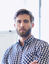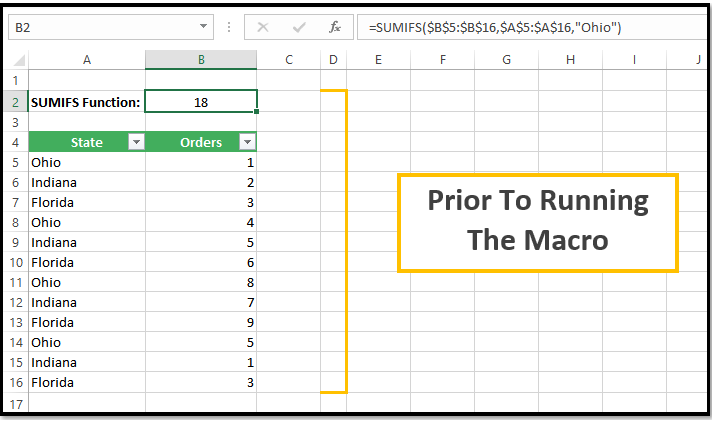Search
•James Richard

# VBA To Show Details For A SUMIFS Function

Updated: Apr 14

### What This VBA Code Does

Someone approached me with code they found on a website that wasn't quite working with their formulas. He asked me to create a VBA macro that would allow him to mimic the double-clicking function of Pivot tables to be able to show the detail of a summarized number, but in this case, for SUMIFS formulas.

The VBA code should run on a single cell’s formula and instantly navigate the user to the source data and filter using just the criteria within the SUMIFS function. This would show the user the detail associated with the results of the SUMIFS function being used within the cell.

The solution proposed in the forum worked for very simplistic formulas with only the use of a single SUMIFS function within the formula and no additional math. For example, the following would work:

=SUMIFS(\$C\$3:\$C\$14,\$B\$3:\$B\$14,"Ohio")

However, this would not work:

=SUMIFS(\$C\$3:\$C\$14,\$B\$3:\$B\$14,"Ohio")/10

The reason for this being the proposed solution was not accurately extracting just the SUMIFS piece from the formula string. I used my limited knowledge of Regular Expressions within my VBA solution to build upon the code and make it more usable.

You simply run it on any selected cell with a formula utilizing the SUMIFS function and it will filter the source data according.

The VBA Code:

sub DetailForSUMIFS()

'PURPOSE: Display Data Making up a SUMIFS result (similar to a Pivot Table Double-Click)

'INSPIRATION: www.mrexcel.com/forum/excel-questions/616156-drilling-down-into-sumifs-formula.html

Dim SumRange As Range

Dim CriteriaRange As Range

Dim CriteriaValue AsVariant

Dim DataSheet As Worksheet

Dim TargetCell As Range

Dim FormulaString AsString

Dim TestExpression As String

Dim objRegEx AsObject

Dim RegExResult AsObject

Dim InputArray AsVariant

Dim x AsInteger

Dim FirstField AsInteger

'Store activecell into a variable

Set TargetCell = ActiveCell

'Ensure cell's formula contains a SUMIFS function

IfNot TargetCell.Formula Like "*SUMIFS(*" Then

MsgBox "No SUMIFS Function reference was found. Aborting..."

ExitSub

EndIf

'Isolate SUMIFS function via REGEX rule

Set objRegEx = CreateObject("VBScript.RegExp")

objRegEx.IgnoreCase = True

objRegEx.Global = True

objRegEx.Pattern = """.*""" ' remove any prior rules

TestExpression = CStr(TargetCell.Formula)

'Isolate anything between "SUMIFS(" and ")"

objRegEx.Pattern = "SUMIFS\((.*?)\)"

'objRegEx.Pattern = "(?<=SUMIFS\()(.*)(?=\))" '>> not sure why this rule does not work...

'Gather Result from RegEx Rule (only using first match)

If objRegEx.test(TestExpression) Then

Set RegExResult = objRegEx.Execute(TestExpression)

If RegExResult.Count > 0 Then

ForEach Match In RegExResult

FormulaString = Match.Value

ExitFor

Next Match

EndIf

Else

ExitSub'RegEx Rule failed to find anything

EndIf

'Split SUMIFS function inputs via "," and store in Array variable

FormulaString = Replace(FormulaString, "SUMIFS(", "")

FormulaString = Left(FormulaString, Len(FormulaString) - 1)

InputArray = Split(FormulaString, ",")

'Determine Range of the first Criteria in formula (2nd Input)

Set CriteriaRange = Range(InputArray(1))

'Pull Sheet Reference for where data is stored from Criteria Range

With CriteriaRange

Set DataSheet = Workbooks(.Parent.Parent.Name).Worksheets(.Parent.Name)

EndWith

'Remove any existing filters on data & turn filtering on (if applicable)

If DataSheet.AutoFilterMode And DataSheet.FilterMode Then

DataSheet.ShowAllData 'Clear Filters

ElseIfNot DataSheet.AutoFilterMode Then

CriteriaRange.CurrentRegion.AutoFilter 'Turn on Filters

EndIf

'Apply SUMIFS Filtering to Source Data

For x = 1 ToUBound(InputArray)

'Ensure we are looking at only Criteria range-related inputs (#2, #4, etc...)

If x Mod 2 <> 0 Then

'Determine Location of first column in Source Data

FirstField = DataSheet.Range(InputArray(x)).Column - DataSheet.AutoFilter.Range.Columns(1).Column + 1

'Determine Criteria Value to Filter Data by

CriteriaValue = Evaluate(InputArray(x + 1))

DataSheet.Range(InputArray(x)).AutoFilter Field:=FirstField, Criteria1:=CriteriaValue

EndIf

Next x

'Store SUMIFS first input (Sum Range) to a variable

Set SumRange = Range(InputArray(0))

'Select the SUM Range to display total amout in Excel's Status Bar

Application.Goto SumRange

'Scroll to the top of the data set

ActiveWindow.ScrollRow = 1

EndSub

## Here is an Example of How This Works:

Here is a simple setup, where the SUMIFS formula is summing all the orders associated with the state of Ohio.After running the macro, you can see that the Ohio data is filtered and the sum range is highlighted. This allows me to look at the bottom right corner of my Excel window and easily see that the sum of the filtered data matches the result my SUMIFS formula is coming up with.## How Do I Modify This To Fit My Specific Needs?

Chances are this post did not give you the exact answer you were looking for. We all have different situations and scenarios and it is impossible to account for every particular one that you might encounter. However, it is always a good start to get you going. If you are looking for an automated tool, that does all of this for you without having to lift a finger, DataRails is the place to check out. It is an FP&A solution made to consolidate data from any existing system into one unified database to give you real-time, accurate insights into your data sets.